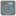• # Some questions about bytecode

## Question related to missionFibonacci Golf

First, I learned a lot from this task. I'm not an experienced python user, and my progression (currently an hardly earned 13rd place for 443 points) have been mostly random.

I don't know much about compiled python code and therefore about how reduce it.

I experimented that most of the time,

```a, b, c = 0, 0, 1
```

takes less bytes than :

```a = 0
b = 0
c = 1
```

but not everytime. Can somebody explain to me why ?

same thing for :

```a = ('s' == 'some_string') # any operation returning a value
b = x * a
c = x + a
```

is sometime less, sometime more than :

```b = x * ('s' == 'some_string')
c = x + ('s' == 'some_string')
```

how can that kind of thing be predicted ?

Many other things questioned me a lot, like is :

```for _ in range (n)
foo()
```

equivalent to :

```while True:
foo()
if i == n: break
i+=1
```

With none of that knowledge I finaly managed to write a 9 lines function with no "if", one "or" a single "while" loop, and 8 comparisons operators, getting points one by one, and i have to admit that this was very challenging for me.

Thank you !16